The intent of this blog is to summarize and document a personal project I did after completion of a “specialization” on Machine Learning on Coursera platform. This blog documents application of Bernoulli Mixture Models to unsupervised learning of handwritten digits dataset.

Reference/Inspiration: Pattern Recognition and Machine Learning by Christopher M. Bishop. Chapter 9, Figure 9.10.
Dataset: MNIST Handwritten Digits.
Model: Bernoulli Mixture Models
Algorithm: Expectation-Maxization

# Mixture of Bernoulli Distribution

First, consider a single multivariate random variable $\mathbf{x}$ with Bernoulli distribution of $D$ independent binary variables $x_i \in \{0, 1\}$, where $i = 1,...,D$, each of which is in turn a univariate Bernoulli distribtion with parameter $\mu_i$,

where, $\mathbf{x} = (x_1,...,x_D)^T$, and $\boldsymbol{\mu} = (\mu_1,...,\mu_D)^T$.

Now consider a finite mixture of $K$ multivariate Bernoulli distributions given by,

$p(\mathbf{x}\,|\, \boldsymbol{\mu}, \boldsymbol{\pi}) = \displaystyle\sum_{k=1}^K \pi_k p(\mathbf{x}\,|\,\boldsymbol{\mu}_k)$ where,

$\boldsymbol\mu = \{\boldsymbol\mu_1,...,\boldsymbol\mu_K\}$ or $% $, $\boldsymbol\pi = \{\pi_1,...,\pi_K\}$, and

Given a data set of $\mathbf{X} = \left\{\mathbf{x}_1,...,\mathbf{x}_N\right\}$, with each observation represented as a mixture of K Bernoulli distributions, then the log likelihood function is:

The appearance of the summation inside the logarithm in the above function means maximixum likelihood solution no longer has a closed form solution.

To maximize the log likelihood function using Expection-Maximization approach, consider an explicit latent variable $\mathbf{z}$ associated with each observation $\mathbf{x}$, where $\mathbf{z}=(z_1,...,z_K)^T$ is a binary K-dimensional variable having a single component equal to 1, and all others set to 0. The latent variable $\mathbf{z}$ can be considered as a membership indicator for each observation. The marginal distribution of $\mathbf{z}$ is specified in terms of the mixing coefficients $\pi_k$, such that $p(z_k = 1) =\,\pi_k$, or $p(\mathbf{z}\,|\,\boldsymbol{\pi}) = \displaystyle\prod_{k=1}^K \pi_k^{z_k}$ We can write the conditional distribution of $\mathbf{x}$, given the latent variable as $p(\mathbf{x}\,|\,\mathbf{z},\boldsymbol{\mu}) = \displaystyle\prod_{k=1}^K\,p(\mathbf{x}\,|\,\boldsymbol{\mu}_k)^{z_k}$

Now formulate the probability of the complete-data (observed $\mathbf{x}$ and latent $\mathbf{z}$) using Bayes’ theorem, $p(\mathbf{x},\mathbf{z}) = p(\mathbf{x}\,|\,\mathbf{z})p(\mathbf{z})$. For the complete-data, the probability is

The corresponding complete-data log likelihood functions is:

Notice that the above log likelihood function can be considered as a linear combination of $z_{nk}$. Since the expectation of a sum is the sum of the expectations, we can write the expectation of the complete-data log likelihood functions with respect to the posterior distribution of the latent variable as:

where, $\gamma(z_{nk}) = \mathbb{E}[z_{nk}]$ is the posterior probability or responsibility of the mixture component $k$ given the data point $x_n$.

## E-M Algorithm for the Mixture of Bernoulli Distributions:

With the above background, the E-M algorithm takes the following form.
E-Step:
Calculation of the responsibilites make the E step of the E-M algorithm.

M-Step:
Maximizing the expectation of the complete-data log likelihood with respect to $\boldsymbol\mu_k$ and $\boldsymbol\pi_k$ yields the M step of the E-M algorithm:

and

where, $N_k\,=\,\displaystyle\sum_{n=1}^N\,\gamma(z_{nk})$

import numpy as np
import matplotlib.pyplot as plt
%matplotlib inline

from sklearn.datasets import fetch_mldata

mnist = fetch_mldata("MNIST original")
mnist.data.shape
'''
MNIST data is in grey scale [0, 255].
Convert it to a binary scale using a threshold of 128.
'''
mnist3 = (mnist.data/128).astype('int')

def show(image):
'''
Function to plot the MNIST data
'''
fig = plt.figure()
imgplot = ax.imshow(image, cmap=plt.cm.Greys)
imgplot.set_interpolation('nearest')
ax.xaxis.set_ticks_position('top')
ax.yaxis.set_ticks_position('left')
plt.show()

def bernoulli(data, means):
'''To compute the probability of x for each bernouli distribution
data = N X D matrix
means = K X D matrix
prob (result) = N X K matrix
'''
N = len(data)
K = len(means)
#compute prob(x/mean)
# prob[i, k] for ith data point, and kth cluster/mixture distribution
prob = np.zeros((N, K))

for i in range(N):
for k in range(K):
prob[i,k] = np.prod((means[k]**data[i])*((1-means[k])**(1-data[i])))

return prob

def respBernoulli(data, weights, means):
'''To compute responsibilities, or posterior probability p(z/x)
data = N X D matrix
weights = K dimensional vector
means = K X D matrix
prob or resp (result) = N X K matrix
'''
#step 1
# calculate the p(x/means)
prob = bernoulli(data, means)

#step 2
# calculate the numerator of the resp.s
prob = prob*weights

#step 3
# calcualte the denominator of the resp.s
row_sums = prob.sum(axis=1)[:, np.newaxis]

# step 4
# calculate the resp.s
try:
prob = prob/row_sums
return prob
except ZeroDivisionError:
print("Division by zero occured in reponsibility calculations!")


def bernoulliMStep(data, resp):
'''Re-estimate the parameters using the current responsibilities
data = N X D matrix
resp = N X K matrix
return revised weights (K vector) and means (K X D matrix)
'''
N = len(data)
D = len(data)
K = len(resp)

Nk = np.sum(resp, axis=0)
mus = np.empty((K,D))

for k in range(K):
mus[k] = np.sum(resp[:,k][:,np.newaxis]*data,axis=0) #sum is over N data points
try:
mus[k] = mus[k]/Nk[k]
except ZeroDivisionError:
print("Division by zero occured in Mixture of Bernoulli Dist M-Step!")
break

return (Nk/N, mus)

def llBernoulli(data, weights, means):
'''To compute expectation of the loglikelihood of Mixture of Beroullie distributions
Since computing E(LL) requires computing responsibilities, this function does a double-duty
to return responsibilities too
'''
N = len(data)
K = len(means)

resp = respBernoulli(data, weights, means)

ll = 0
for i in range(N):
sumK = 0
for k in range(K):
try:
temp1 = ((means[k]**data[i])*((1-means[k])**(1-data[i])))
temp1 = np.log(temp1.clip(min=1e-50))

except:
print("Problem computing log(probability)")
sumK += resp[i, k]*(np.log(weights[k])+np.sum(temp1))
ll += sumK

return (ll, resp)

def mixOfBernoulliEM(data, init_weights, init_means, maxiters=1000, relgap=1e-4, verbose=False):
'''EM algo fo Mixture of Bernoulli Distributions'''
N = len(data)
D = len(data)
K = len(init_means)

#initalize
weights = init_weights[:]
means = init_means[:]
ll, resp = llBernoulli(data, weights, means)
ll_old = ll

for i in range(maxiters):
if verbose and (i % 5 ==0):
print("iteration {}:".format(i))
print("   {}:".format(weights))
print("   {:.6}".format(ll))

#E Step: calculate resps
#Skip, rolled into log likelihood calc
#For 0th step, done as part of initialization

#M Step
weights, means = bernoulliMStep(data, resp)

#convergence check
ll, resp = llBernoulli(data, weights, means)
if np.abs(ll-ll_old)<relgap:
print("Relative gap:{:.8} at iternations {}".format(ll-ll_old, i))
break
else:
ll_old = ll

return (weights, means)

from sklearn.utils import shuffle

def pickData(digits, N):
sData, sTarget = shuffle(mnist3, mnist.target, random_state=30)
returnData = np.array([sData[i] for i in range(len(sData)) if sTarget[i] in digits])
return shuffle(returnData, n_samples=N, random_state=30)

def experiments(digits, K, N, iters=50):
'''
Picks N random points of the selected 'digits' from MNIST data set and
fits a model using Mixture of Bernoulli distributions.
And returns the weights and means.
'''

expData = pickData(digits, N)

D = len(expData)

initWts = np.random.uniform(.25,.75,K)
tot = np.sum(initWts)
initWts = initWts/tot

#initMeans = np.random.rand(10,D)
initMeans = np.full((K, D), 1.0/K)

return mixOfBernoulliEM(expData, initWts, initMeans, maxiters=iters, relgap=1e-15)

finWeights, finMeans = experiments([2,3,9], 3, 1000)
[show(finMeans[i].reshape(28,28)) for i in range(len(finMeans))]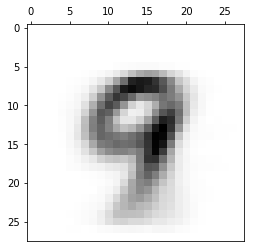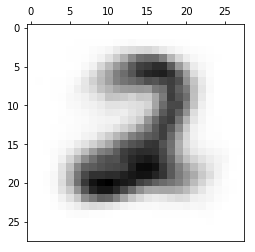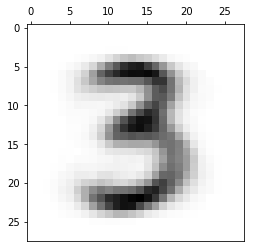[None, None, None]

finWeights, finMeans = experiments([1,2,3,7], 4, 5000)
[show(finMeans[i].reshape(28,28)) for i in range(len(finMeans))]
print(finWeights)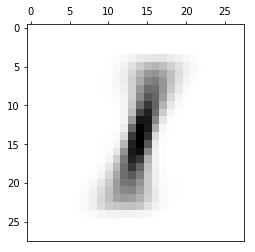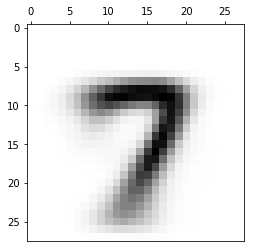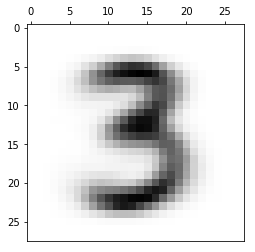[ 0.26757071  0.24729179  0.23207817  0.25305934]

finWeights, finMeans = experiments([2,3,7], 4, 3000)
[show(finMeans[i].reshape(28,28)) for i in range(len(finMeans))]
print(finWeights)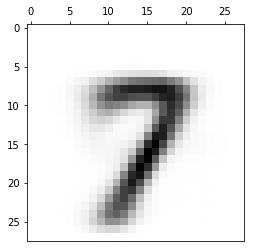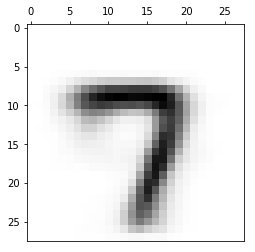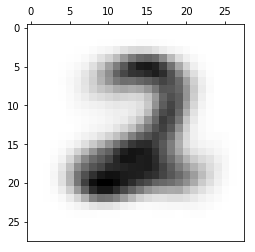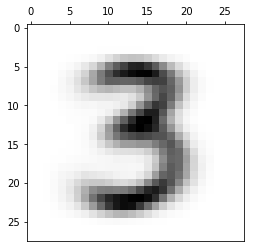[ 0.1912005   0.15238828  0.34022079  0.31619044]